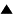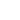# Multiple axiomatization sets, a metaphor for metafoundationalism

A simple metaphor for our understanding of metafoundationalism can be found in mathematics. Mathematical systems are defined by a set of axioms, from which theorems are deduced. A mathematical theory might be defined as the set of all propositions that are true under the given set of axioms (theorems). For example, a theory of addition would contain propositions like "1 + 2 = 3", "2 + 5 = 3 + 4", and axioms like "a + b = b + a", "a + 0 = a", etc. Now, it is a well-known fact that in general the same theory can be generated by multiple sets of axioms. For example, the Boolean logic of propositions has many different axiomatizations which are formally equivalent (producing the same theorems), though one may be preferred to the other on the grounds of simplicity, esthetic appeal or explicitness.

Similarly, a metafoundational theory would consist of a set of propositions which can be derived from multiple sets of foundational propositions. Propositions which are primary (axioms) in one system would be derived in another system. No set of fundamental propositions would be absolutely primary. The whole theory should rather be viewed as a bootstrapping (cf. Heylighen's paper on "Knowledge structuring") network, where A derives from B and B derives from A.

A mathematical structure which might possibly express this arrangement is a multiply rooted DAG (Directed Acyclic Graph).

 HomeMetasystem Transition TheoryMethodology for the Development of MSTTMultiple Beginnings, Meta-Foundationalism Up Prev.Next Down